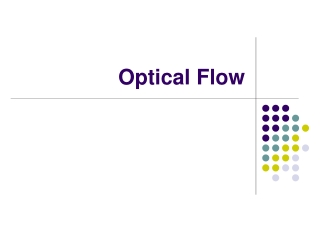DownloadDownload PresentationOptical Flow

# Optical Flow

Télécharger la présentation## Optical Flow

- - - - - - - - - - - - - - - - - - - - - - - - - - - E N D - - - - - - - - - - - - - - - - - - - - - - - - - - -
##### Presentation Transcript

1. Optical Flow

2. Optical Flow • Brightness Constancy • The Aperture problem • Regularization • Lucas-Kanade • Coarse-to-fine • Parametric motion models • Direct depth • SSD tracking • Robust flow • Textured motion

3. Optical Flow:Where do pixels move to?

4. Motion is a basic cue Motion can be the only cue for segmentation

5. Applications • tracking • structure from motion • motion segmentation • stabilization • compression • mosaicing • …

6. Optical Flow • Brightness Constancy • The Aperture problem • Regularization • Lucas-Kanade • Coarse-to-fine • Parametric motion models • Direct depth • SSD tracking • Robust flow • Textured motion

7. Definition of optical flow OPTICAL FLOW = apparent motion of brightness patterns Ideally, the optical flow is the projection of the three-dimensional velocity vectors on the image 

8. Caution required ! Two examples : 1. Uniform, rotating sphere  O.F. = 0 2. No motion, but changing lighting  O.F.  0 

9. Mathematical formulation I (x,y,t) = brightness at (x,y) at time t Brightness constancy assumption: Optical flow constraint equation : 

10. Optical Flow • Brightness Constancy • The Aperture problem • Regularization • Lucas-Kanade • Coarse-to-fine • Parametric motion models • Direct depth • SSD tracking • Robust flow • Textured motion

11. The aperture problem 1 equation in 2 unknowns 

12. What is Optical Flow, anyway? • Estimate of observed projected motion field • Not always well defined! • Compare: • Motion Field (or Scene Flow) projection of 3-D motion field • Normal Flow observed tangent motion • Optical Flow apparent motion of the brightness pattern (hopefully equal to motion field) • Consider Barber pole illusion

13. Optical Flow • Brightness Constancy • The Aperture problem • Regularization • Lucas-Kanade • Coarse-to-fine • Parametric motion models • Direct depth • SSD tracking • Robust flow • Textured motion

14. Horn & Schunck algorithm Additional smoothness constraint : besides OF constraint equation term minimize es+ec 

15. so the Euler-Lagrange equations are is the Laplacian operator Horn & Schunck The Euler-Lagrange equations : In our case , 

16. Horn & Schunck Remarks : 1. Coupled PDEs solved using iterative methods and finite differences 2. More than two frames allow a better estimation of It 3. Information spreads from corner-type patterns 

17. Horn & Schunck, remarks 1. Errors at boundaries 2. Example of regularization (selection principle for the solution of ill-posed problems) 

18. Results of an enhanced system

19. Structure from motion with OF

20. Optical Flow • Brightness Constancy • The Aperture problem • Regularization • Lucas-Kanade • Coarse-to-fine • Parametric motion models • Direct depth • SSD tracking • Robust flow • Textured motion

21. Optical Flow • Brightness Constancy • The Aperture problem • Regularization • Lucas-Kanade • Coarse-to-fine • Parametric motion models • Direct depth • SSD tracking • Robust flow • Textured motion

22. Optical Flow • Brightness Constancy • The Aperture problem • Regularization • Lucas-Kanade • Coarse-to-fine • Parametric motion models • Direct depth • SSD tracking • Robust flow • Textured motion